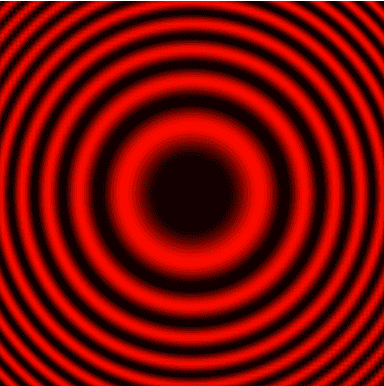# Constructive, destructive interference help please

SHAWN JAMES
Homework Statement:
One of the beams of an interferometer passes through a small evacuated glass container 1.155 cm deep. When a gas is allowed to slowly fill the container, a total of 177 dark fringes are counted to move past a reference line. The light used has a wavelength of 632.1 nm .
Relevant Equations:
n(gas) = 1 + [m(lambaair)]/(2*t)
hi,
i understand how to get to this equation, and this is the equation that gives the answer. However, the question asks for dark fringes which is destructive interference? would you not use m/2? so instead of the denominator (2*t) would it not be (4*t)?

Homework Helper
Gold Member
Can you solve the problem if, instead of dark fringes, 177 bright fringes went past the reference line? If so, don't you get a bright fringe to go by for every dark fringe that goes by?

SHAWN JAMES
yes I guess you could solve for bright fringes but why would use bright fringes instead of dark fringes when the question tells you about the dark fringes?

Homework Helper
Gold Member
yes I guess you could solve for bright fringes but why would use bright fringes instead of dark fringes when the question tells you about the dark fringes?
You missed my point because apparently you do not really understand the relevant equation that you posted in #1. Please explain to me what ##m## stands for in that equation and why one must divide it by 2 if one counts dark as opposed to bright fringes go by reference line.

SHAWN JAMES
i'm honestly not sure why one divides by 2. but m stands for fringes and for dark fringes you divide by 2?

Homework Helper
Gold Member
OK, you said you understand how to get this equation. Can you explain it to me in your own words? Start with how an interferometer can be used to measure the index of refraction of a gas and how collected information needs to be processed. You might find https://opentextbc.ca/universityphysicsv3openstax/chapter/the-michelson-interferometer/ useful. Post your explanation when you're ready.

SHAWN JAMES
One beam travels twice through the glass container of thickness t. thus corresponds to 2t/λgas wavelengths. The other beam travels through an equal amount of air, but because of the different index this corresponds to 2t/λair wavelengths. The difference in these is the cause of the shift in fringes. So it becomes m/2=2t/λgas-2t/λair. This is how I derived the equation, and since it gives you the wavelength in air not in the λgas you have to use ngas*λgas= nair*λair and replace λgas to solve for ngas but I'm still confused as to why you don't use m/2.

SHAWN JAMES
helloooo?

Homework Helper
Gold Member
Good, but not perfect. You understand the principle of using the interferometer to measure the index of refraction. Just put together what you understand and forget that you are counting dark or bright fringes. If the gas is not there, the number of wavelengths is ## N_{air}=\dfrac{2t}{\lambda}##; if the gas is there, the number of wavelengths is ## N_{gas}=\dfrac{2t}{\lambda/n}##. You know that if you increase the length of one of the interferometer arms by one wavelength, you get the same pattern except that the fringes have shifted by one relative to a fixed point. If you increase the number of wavelengths from ##N_{air}## without the gas to ##N_{gas}## with the gas ##\dots##

SHAWN JAMES
i'm sorry for my stupidity, but I'm still confused.

Homework Helper
Gold Member
Perhaps you are confused because you do not understand what is going on physically with an interferometer. The fringes you see look like this. Note the central dark circle. It shows destructive interference at the center.When you start moving one arm slowly (say you make it shorter), you will see the fringes shrink towards the center. When the arm has moved by a quarter wavelength (round trip of half wavelength), you will have destructive interference. This means a bright spot at the center of the pattern created by the shrinking of the first ring. All dark areas are replaced by bright areas. If you keep on moving the arm in the same direction, eventually the pattern will look the same as in the picture, except that the first ring has disappeared while ring 2 became ring 1, ring 3 became ring 2 and so on. If you imagine a fixed reference line drawn on the screen, one fringe has moved past it. This happens when the round trip distance traveled by the light along the arm has changed by one wavelength.

So changing the light's path length by one wavelength corresponds to one fringe moving past the reference line. If you have 177 fringes moving past, you have light traveling an additional distance equal to 177 wavelengths more than the other arm. So if your units of distance are wavelengths the optical path length difference is 177.

In post #9 I gave you the number of wavelengths in the length ##2t## for each arm. Can you construct a formula relating these to 177? If not, let me know.

•etotheipi and hutchphd